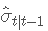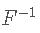# 介绍

## 数据

```

# 加载库
library(tidyverse)

# 加载数据

# 每只股票一栏

plot( y = stok\$C , geo = 'line')```

```ret = diff(stoks\$C) / socs\$C\[-legth\]

plot(x = 1:length, y = res )```

`adf.test(ret)`

# Python计算股票投资组合的风险价值（VaR）

### 识别

• ∑u^2= 残差平方和
• T = 观察次数
• k = 模型参数的数量 (p + q + 1)

### 随时关注您喜欢的主题

`auto.arima(rets )`

### 估计

`model`

rt=0.0437∗rt−1−0.0542∗rt−2+ϵt 其中 ϵt 是白噪声

### 诊断检查

`ggtsdisplay(plot)`

ACF 和 PACF 图很相似，自相关似乎为零。右下角图表示残差与正态分布 N(0, σ2σ2) 相比的直方图。

# 为了进一步检验残差不相关的假设，我们执行 Ljung-Box 检验。

QLB统计量不对称地遵循具有 mpq 自由度的 X2 分布。零假设是指 H0:ρ1=ρ2=⋯=ρm=0

`Box.test(resiuas)`

# 当残差平方相关时，GARCH 过程有效。ACF 和 PACF 图清楚地表明显着相关性。

```#模型定义
ugarchpec(varin ,
mean.model

fit(sec = model.spec ')```

a1和 β1都显着不同于零，因此假设残差随时间变化的波动率是合理的。

σt−12 项的连续替换，GARCH 方程可以写为：

## 风险价值

VaR 统计具有三个组成部分：a) 时间段，b) 置信水平，c) 损失金额（或损失百分比）。对于 95% 的置信水平，我们可以说最坏的每日损失不会超过 VaR 估计。如果我们使用历史数据，我们可以通过取 5% 的分位数值来估计 VaR。对于我们的数据，这个估计是：

`quante(res , 0.05)`
`qplot(ret)`

## 分布特征

`ja.tst(rets)`
`qplot(ret , gom = 'desity') + geom_density`

`fitdispars`
```cat("对于 a = 0.05，正态分布的分位数值为：" ,
qnorm(p = 0.05) , "\\n" ,
)```

## Garch VaR 和Delta-normal 方法

Delta-normal 方法假设所有股票收益都是正态分布的。这种方法包括回到过去并计算收益的方差。风险价值可以定义为：是给定 t−1 信息的条件标准偏差，并且是 t 分布的逆 PDF 函数。

`qplot(y = rets , gom = 'point') + gem_pnt(col = 'lihtgrson')`

## VaR预测

```roll = garhrol(spec = model.spec )

#测试集 500 个观察
mean(ret) + rolldesiy\[,'Siga'\]*qdist(dis='std',```

## 回测

```for(i in 1:50 p\[i\]= (pbinom(q = (i-1 ) - pbinom(q ))

qplot(y = p )```

```plot(VaR95, geom = 'line') +
geom_point```

`cat('delta-normal 方法的异常数：', (sum(rets\[759:1258\] < (mean(r\]`

## 参考

Angelidis T., Benos A. and Degiannakis S. (December 2003). The Use of GARCH Models in VaR Estimation.

Kaizong Ye拓端研究室（TRL）的研究员。

​非常感谢您阅读本文，如需帮助请联系我们！QQ在线咨询

15121130882

0571-63341498

## 关注有关新文章的微信公众号

This will close in 0 seconds